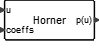# Horner

This block evaluates a polynomial using the Horner method. Polynomial coefficients may be constant and given as block parameters or variable given by the second input.MathOperations

## Description

The Horner block evaluates a polynomial where the argument is an input and the result of the evaluation, the output. The polynomial coefficients may be given as a vector block parameter or as a second input vector. The coefficients of the polynomial are arranged in the vector in descending order (the last vector entry thus corresponding to the constant term). The argument may be a matrix. The polynomial is evaluated for each element of the input matrix separately producing as output a matrix of identical size. Double and complex data types are supported.

## ParametersNameLabelDescriptionData TypeValid Values

mode

Polynomial coefficients given as parameter

Number

0
1

coeffs

Polynomial coefficients

Vector

## Ports

NameTypeDescriptionIO TypeNumber

u

explicit

input

1

Port 2

explicit

input

1-mode

p(u)

explicit

output

1